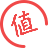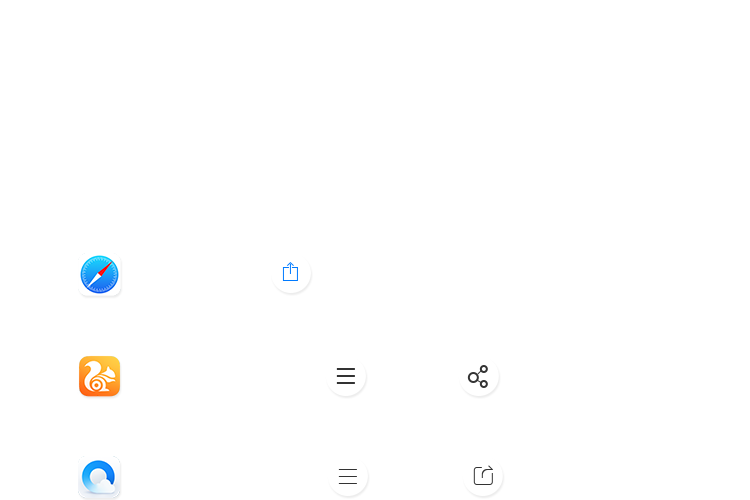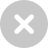# 用你的Excel打造一个随机密码生成器11-06 21:10 + 关注>>>创建随机数字>>>创建随机字母>>>创建包含随机字母、数字与字符的密码>>>创建随机长度且包含随机字母、数字与字符的密码

`Public` `Function` `RandomizeF(Num1 ``As` `Integer``, Num2 ``As` `Integer``)`

`Dim` `Rand ``As` `String`

`Application.Volatile`

`getLen = Int((Num2 + 1 - Num1) * Rnd + Num1)`

`Do`

` ``i = i + 1`

` ``Randomize`

` ``Rand = Rand & Chr(Int((85) * Rnd + 38))`

`Loop` `Until` `i = getLen`

`RandomizeF = Rand`

`End` `Function`4
16
0